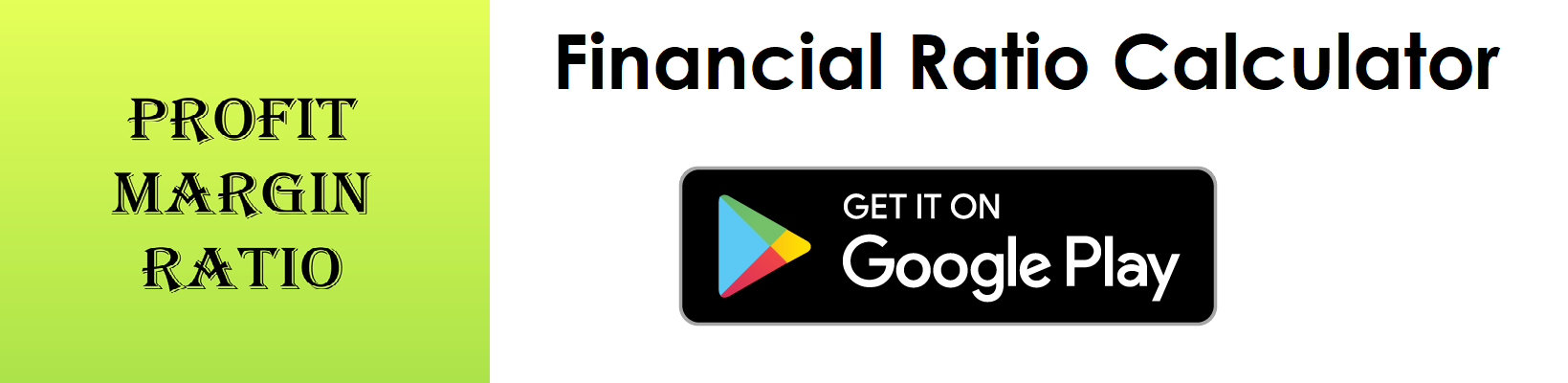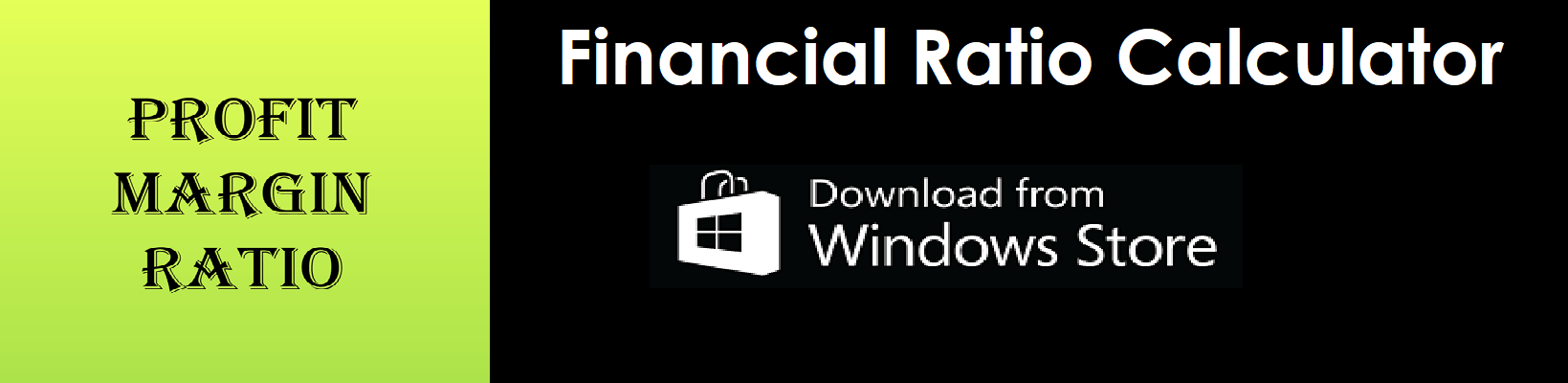# Debt Ratio Calculator

Key in the Total Liabilities and Total Assets Values from your Balance Sheet to the respective fields given below and then click Calculate to get the desired result.

##### Debt Ratio Calculator - Glossary:
Using our debt ratio calculator, helps to compare, measure, understand the overall health of the business.

##### Debt Ratio:
Debt ratio are used to compare debt (total liabilities) to its total assets. It shows proportion of a company's assets that financed by debt.

##### How to calculate?
Formula: Debt Ratio = Total Liabilities/Total Assets
This is a balance sheet component; the values are commonly stated against Total Liabilities and Total Assets.

##### Total Liabilities:
Total Liabilities is the source of the funds such as short term and long term external borrowing that is mainly used to fund its asset purchase.

##### Total Assets:
Total assets are the sum of all current and noncurrent assets that a company owns.

Example:
Debt ratio for a company with a total liability of \$30,000 and total assets of \$100,000 is 0.36:1. It means that, 36% of its assets are financed by debt.

# Download our Mobile Apps - today.